Example 54.13 Complementary Log-Log Model for Infection Rates

Antibodies produced in response to an infectious disease like malaria remain in the body after the individual has recovered from the disease. A serological test detects the presence or absence of such antibodies. An individual with such antibodies is called seropositive. In geographic areas where the disease is endemic, the inhabitants are at fairly constant risk of infection. The probability of an individual never having been infected in Y years is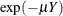, whereis the mean number of infections per year (see the appendix of Draper, Voller, and Carpenter 1972). Rather than estimating the unknown, epidemiologists want to estimate the probability of a person living in the area being infected in one year. This infection rateis given by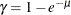The following statements create the data set sero, which contains the results of a serological survey of malarial infection. Individuals of nine age groups (Group) were tested. The variable A represents the midpoint of the age range for each age group. The variable N represents the number of individuals tested in each age group, and the variable R represents the number of individuals that are seropositive.

data sero;
input Group A N R;
X=log(A);
label X='Log of Midpoint of Age Range';
datalines;
1  1.5  123  8
2  4.0  132  6
3  7.5  182 18
4 12.5  140 14
5 17.5  138 20
6 25.0  161 39
7 35.0  133 19
8 47.0   92 25
9 60.0   74 44
;

For the ith group with the age midpoint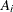, the probability of being seropositive is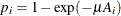. It follows that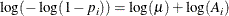By fitting a binomial model with a complementary log-log link function and by using X=log(A) as an offset term, you can estimate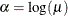as an intercept parameter. The following statements invoke PROC LOGISTIC to compute the maximum likelihood estimate of. The LINK=CLOGLOG option is specified to request the complementary log-log link function. Also specified is the CLPARM=PL option, which requests the profile-likelihood confidence limits for.

proc logistic data=sero;
model R/N= / offset=X
clparm=pl
scale=none;
title 'Constant Risk of Infection';
run;

Results of fitting this constant risk model are shown in Output 54.13.1.

Output 54.13.1: Modeling Constant Risk of Infection

 Constant Risk of Infection

The LOGISTIC Procedure

Model Information
Data Set WORK.SERO
Response Variable (Events) R
Response Variable (Trials) N
Offset Variable X Log of Midpoint of Age Range
Model binary cloglog
Optimization Technique Fisher's scoring

 Number of Observations Read 9 9 1175 1175

Response Profile
Ordered
Value
Binary Outcome Total
Frequency
1 Event 193
2 Nonevent 982

Intercept-Only Model Convergence Status
Convergence criterion (GCONV=1E-8) satisfied.

-2 Log L = 967.1158

Deviance and Pearson Goodness-of-Fit Statistics
Criterion Value DF Value/DF Pr > ChiSq
Deviance 41.5032 8 5.1879 <.0001
Pearson 50.6883 8 6.3360 <.0001

Number of events/trials observations: 9

Analysis of Maximum Likelihood Estimates
Parameter DF Estimate Standard
Error
Wald
Chi-Square
Pr > ChiSq
Intercept 1 -4.6605 0.0725 4133.5626 <.0001
X 0 1.0000 0 . .

Parameter Estimates and Profile-Likelihood
Confidence Intervals
Parameter Estimate 95% Confidence Limits
Intercept -4.6605 -4.8057 -4.5219

Output 54.13.1 shows that the maximum likelihood estimate ofand its estimated standard error are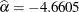and, respectively. The infection rate is estimated as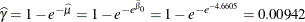The 95% confidence interval for, obtained by back-transforming the 95% confidence interval for, is (0.0082, 0.0108); that is, there is a 95% chance that, in repeated sampling, the interval of 8 to 11 infections per thousand individuals contains the true infection rate.

The goodness-of-fit statistics for the constant risk model are statistically significant (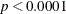), indicating that the assumption of constant risk of infection is not correct. You can fit a more extensive model by allowing a separate risk of infection for each age group. Suppose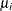is the mean number of infections per year for the ith age group. The probability of seropositive for the ith group with the age midpointis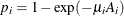, so that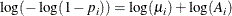In the following statements, a complementary log-log model is fit containing Group as an explanatory classification variable with the GLM coding (so that a dummy variable is created for each age group), no intercept term, and X=log(A) as an offset term. The ODS OUTPUT statement saves the estimates and their 95% profile-likelihood confidence limits to the ClparmPL data set. Note that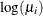is the regression parameter associated with Group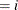.

proc logistic data=sero;
ods output ClparmPL=ClparmPL;
class Group / param=glm;
model R/N=Group / noint
offset=X
clparm=pl;
title 'Infectious Rates and 95% Confidence Intervals';
run;

Results of fitting the model with a separate risk of infection are shown in Output 54.13.2.

Output 54.13.2: Modeling Separate Risk of Infection

 Infectious Rates and 95% Confidence Intervals

The LOGISTIC Procedure

Analysis of Maximum Likelihood Estimates
Parameter   DF Estimate Standard
Error
Wald
Chi-Square
Pr > ChiSq
Group 1 1 -3.1048 0.3536 77.0877 <.0001
Group 2 1 -4.4542 0.4083 119.0164 <.0001
Group 3 1 -4.2769 0.2358 328.9593 <.0001
Group 4 1 -4.7761 0.2674 319.0600 <.0001
Group 5 1 -4.7165 0.2238 443.9920 <.0001
Group 6 1 -4.5012 0.1606 785.1350 <.0001
Group 7 1 -5.4252 0.2296 558.1114 <.0001
Group 8 1 -4.9987 0.2008 619.4666 <.0001
Group 9 1 -4.1965 0.1559 724.3157 <.0001
X   0 1.0000 0 . .

Parameter Estimates and Profile-Likelihood
Confidence Intervals
Parameter   Estimate 95% Confidence Limits
Group 1 -3.1048 -3.8880 -2.4833
Group 2 -4.4542 -5.3769 -3.7478
Group 3 -4.2769 -4.7775 -3.8477
Group 4 -4.7761 -5.3501 -4.2940
Group 5 -4.7165 -5.1896 -4.3075
Group 6 -4.5012 -4.8333 -4.2019
Group 7 -5.4252 -5.9116 -5.0063
Group 8 -4.9987 -5.4195 -4.6289
Group 9 -4.1965 -4.5164 -3.9037

For the first age group (Group=1), the point estimate of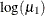is –3.1048, which transforms into an infection rate of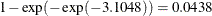. A 95% confidence interval for this infection rate is obtained by transforming the 95% confidence interval for. For the first age group, the lower and upper confidence limits are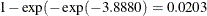and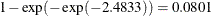, respectively; that is, there is a 95% chance that, in repeated sampling, the interval of 20 to 80 infections per thousand individuals contains the true infection rate. The following statements perform this transformation on the estimates and confidence limits saved in the ClparmPL data set; the resulting estimated infection rates in one year’s time for each age group are displayed in Table 54.18. Note that the infection rate for the first age group is high compared to that of the other age groups.

data ClparmPL;
set ClparmPL;
Estimate=round( 1000*( 1-exp(-exp(Estimate)) ) );
LowerCL =round( 1000*( 1-exp(-exp(LowerCL )) ) );
UpperCL =round( 1000*( 1-exp(-exp(UpperCL )) ) );
run;

Table 54.18: Infection Rate in One Year

Number Infected per 1,000 People

Age

Point

95% Confidence Limits

Group

Estimate

Lower

Upper

1

44

20

80

2

12

5

23

3

14

8

21

4

8

5

14

5

9

6

13

6

11

8

15

7

4

3

7

8

7

4

10

9

15

11

20